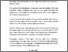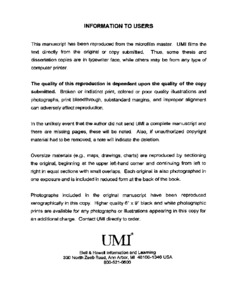Title:

# Necessary and sufficient conditions so that a commutative ring can be embedded into a strongly pi-regular ring

Philippoussis, Anthony (1998) Necessary and sufficient conditions so that a commutative ring can be embedded into a strongly pi-regular ring. Masters thesis, Concordia University.Preview Text (application/pdf) MQ39934.pdf 1MB

## Abstract

If R is commutative ring then R can be embedded into a strongly $\pi$-regular ring if and only if there exists a set of prime ideals Y = $\{$P$\sb\alpha\}\alpha\in\Lambda$ and for each P$\sb\alpha$, a P$\sb\alpha$-primary ideal Q$\sb\alpha$ such that: (I) Y is closed in the patch topology on Spec R. (II) $\rm\cap\sb{\alpha\in\Lambda}Q\sb\alpha=\{0\}$. (III) for each a $\in$ R there is n(a) $\in$ N such that for n $\ge$ n(a), $\rm\{P\sb\alpha\mid P\sb\alpha\in Y$ and $\rm a\sp{n}\in Q\sb\alpha\}$ is patch open in Y.

Divisions: Concordia University > Faculty of Arts and Science > Mathematics and Statistics Thesis (Masters) Philippoussis, Anthony v, 41 leaves ; 29 cm. Concordia University M.Sc. Mathematics 1998 Raphael, Robert QA 251.3 P45 1998 484 Concordia University Library 27 Aug 2009 17:12 13 Jul 2020 19:46 https://concordiauniversity.on.worldcat....
All items in Spectrum are protected by copyright, with all rights reserved. The use of items is governed by Spectrum's terms of access.

Repository Staff Only: item control pageResearch related to the current document (at the CORE website)
Back to top